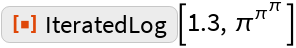#Function Repository Resource:

# IteratedLog

Determine the iterated logarithm of an input

Contributed by: Wolfram|Alpha Math Team
 ResourceFunction["IteratedLog"][z] gives the iterated natural logarithm of z. ResourceFunction["IteratedLog"][b,z] gives the iterated logarithm base b of z.

## Details and Options

The iterated logarithm is also known as inverse tetration or the super-logarithm. It is defined to be the smallest (integer) number of times that the logarithm must be applied to a number to yield a result less than 1.

## Examples

### Basic Examples

IteratedLog is the inverse of tetration (repeated exponentiation):

 In:=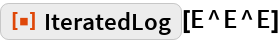Out=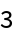A slightly larger input shows a step-like jump in the value of IteratedLog:

 In:=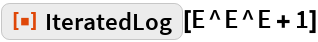Out=Make a table of the iterated logarithm of the first 50 integers:

 In:=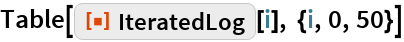Out=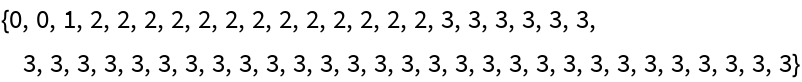### Scope

The logarithmic base can be any real number greater than 1:

 In:=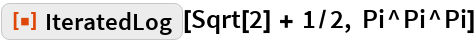Out=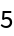### Applications

Plot the iterated logarithm for different logarithmic bases:

 In:=Out=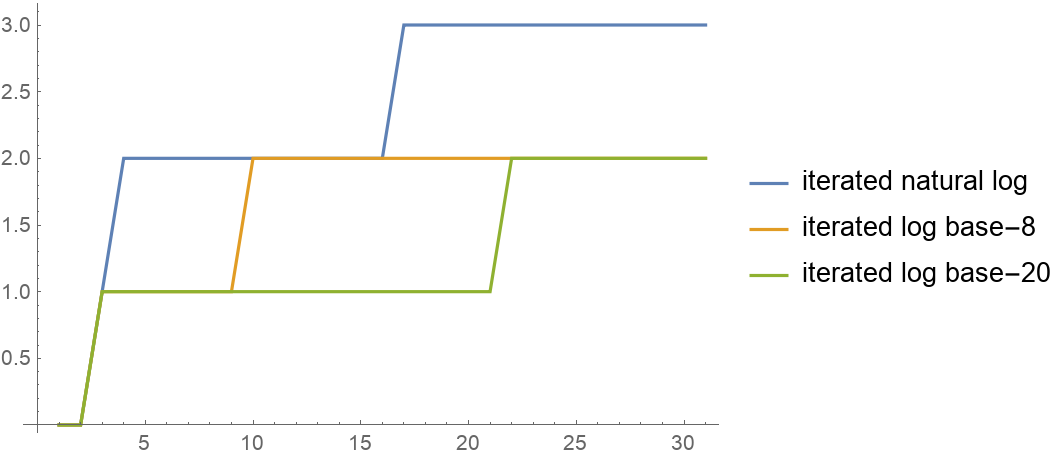### Possible Issues

IteratedLog will return unevaluated in cases where evaluation might lead to numerical overflow:

 In:=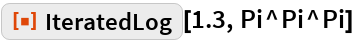Out=